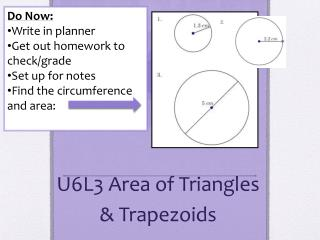DownloadDownload PresentationU6L3 Area of Triangles & Trapezoids

# U6L3 Area of Triangles & Trapezoids

Télécharger la présentation## U6L3 Area of Triangles & Trapezoids

- - - - - - - - - - - - - - - - - - - - - - - - - - - E N D - - - - - - - - - - - - - - - - - - - - - - - - - - -
##### Presentation Transcript

1. Do Now: • Write in planner • Get out homework to check/grade • Set up for notes • Find the circumference and area: U6L3 Area of Triangles & Trapezoids

2. Area of a Triangle • The area of a triangle is half the product of its base b and its height h.

3. Triangles • A diagonal of a parallelogram divides the parallelogram into 2 congruent triangles. • The area of each triangle is half the area of the parallelogram • The base of the triangle can be any side. • The height is the perpendicular distance from the base to the opposite vertex.

4. Practice **Hint**: multiplying by ½ is the same as dividing by 2

5. Area of trapezoids • The area of a trapezoid is half its height multiplied by the sum of the lengths of its two bases

6. Trapezoids • A parallelogram can be divided into 2 congruent trapezoids. • The two parallel sides of a trapezoid are its bases • A = ½ (b1 + b2)h

7. Challenge Problem • Find the missing measurement of each triangle. • b = 12inh = ?A = 42 42 = ½ b(12) 42 = b6 6 = b

8. Assignment • P. 436-437 #7-9, 13-15, 25